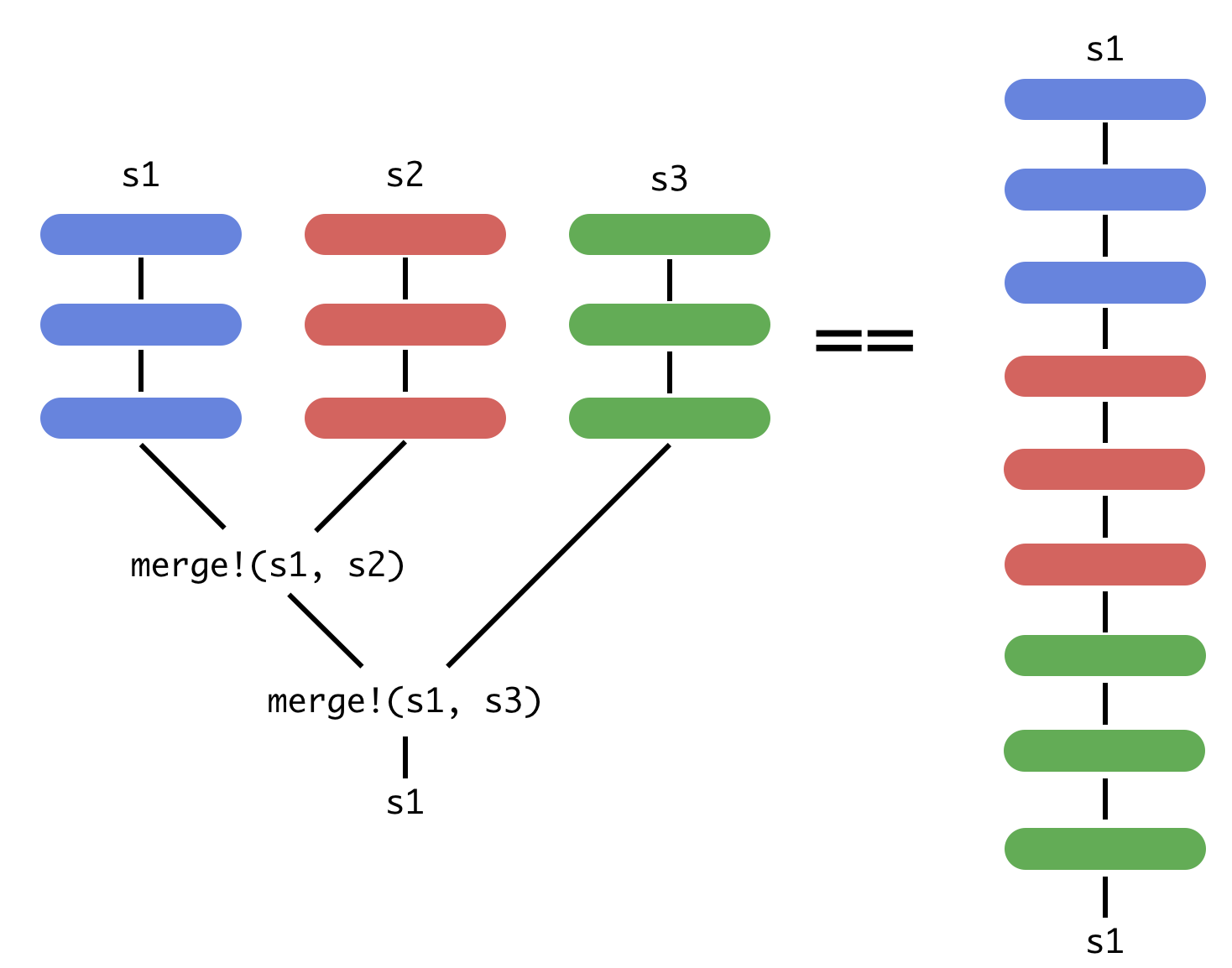OnlineStats Integration

# OnlineStats Integration

OnlineStats is a package for calculating statistics and models with online (one observation at a time) parallelizable algorithms. This integrates tightly with JuliaDB's distributed data structures to calculate statistics on large datasets. The full documentation for OnlineStats is available here.

## Basics

OnlineStats' objects can be updated with more data and also merged together. The image below demonstrates what goes on under the hood in JuliaDB to compute a statistic `s` in parallel.OnlineStats integration is available via the `reduce` and `groupreduce` functions. An OnlineStat acts differently from a normal reducer:

• Normal reducer `f`: `val = f(val, row)`
• OnlineStat reducer `o`: `fit!(o, row)`
``````julia> using JuliaDB, OnlineStats

julia> t = table(1:100, rand(Bool, 100), randn(100));

julia> reduce(Mean(), t; select = 3)
Mean: n=100 | value=0.159962

julia> grp = groupreduce(Mean(), t, 2; select=3)
Table with 2 rows, 2 columns:
1      2
────────────────────────────────────
false  Mean: n=38 | value=-0.0610545
true   Mean: n=62 | value=0.295423

julia> select(grp, (1, 2 => value))
Table with 2 rows, 2 columns:
1      2
─────────────────
false  -0.0610545
true   0.295423``````
Note

The `OnlineStats.value` function extracts the value of the statistic. E.g. `value(Mean())`.

### Calculating Statistics on Multiple Columns.

The `OnlineStats.Group` type is used for calculating statistics on multiple data streams. A `Group` that computes the same `OnlineStat` can be created through integer multiplication:

``reduce(3Mean(), t)``
``````Group
├── Mean: n=100 | value=50.5
├── Mean: n=100 | value=0.62
└── Mean: n=100 | value=0.159962``````

Alternatively, a `Group` can be created by providing a collection of `OnlineStat`s.

``reduce(Group(Extrema(Int), CountMap(Bool), Mean()), t)``
``````Group
├── Extrema: n=100 | value=(1, 100)
├── CountMap: n=100 | value=OrderedCollections.OrderedDict(false=>38,true=>62)
└── Mean: n=100 | value=0.159962``````